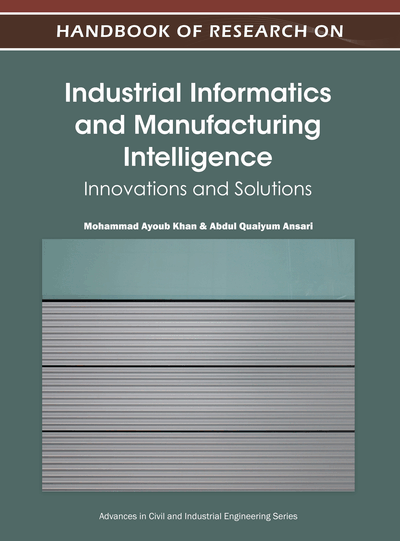# Novel Meta-Heuristic Optimization Techniques for Solving Fuzzy Programming Problems

Pandian Vasant (Universiti Teknologi PETRONAS, Malaysia)
DOI: 10.4018/978-1-4666-0294-6.ch005
Available
\$33.75
List Price: \$37.50
10% Discount:-\$3.75
TOTAL SAVINGS: \$3.75

## Abstract

In this chapter, three meta-heuristic optimization techniques have been utilized to solve the fuzzy programming problems in industrial production systems. This chapter outlines an introduction to real-world industrial problem for product-mix selection involving eight variables and 21 constraints with fuzzy technological coefficients and thereafter, a formulation for an optimization approach to solve the problem. This problem occurs in production planning in which a decision maker plays a pivotal role in making decision under fuzzy environment. Decision-maker should be aware of his/her level of satisfaction as well as degree of fuzziness while making the product-mix decision. Genetic algorithms, pattern search, and mesh adaptive direct search methods have been employed to solve the large scale problems in real world industrial sector. The results for these techniques have been investigated thoroughly in the form of 2D, 3D plots, and tables. The industrial production planning problem which was illustrated in this chapter was solved successfully by these three meta-heuristic methods. The results are analyzed along with the optimal profit function (objective or fitness function) with level of satisfaction, decision variables, vagueness factor, and computational time (CPU).
Chapter Preview
Top

## Introduction

It is well known that optimization problems arise in a variety of situations. Particularly interesting are those concerning management problems as decision makers usually state their data in a vague way: “high demand”, “low supply”, “average price”, etc. Because of this vagueness, managers prefer to have not just one solution but a set of them, so that the most suitable solution can be applied according to the state of existing decision of the production process at a given time and without increasing delay. In these situations fuzzy optimization is an ideal methodology, since it allows us to represent the underlying uncertainty of the meta-heuristic optimization problem, while finding optimal solutions that reflect such uncertainty and then applying them to possible instances, once the uncertainty has been solved. This allows us to obtain a model of the behaviour of the solutions based on the uncertainty of the meta-heuristic optimization problem.

Fuzzy constrained optimization problems have been extensively studied since the seventies. In the linear case, the first approaches to solve the so-called fuzzy linear programming problem appeared in Bellman and Zadeh (1970). Since then, important contributions solving different linear models have been made and these models have been the subject of a substantial amount of work. In the nonlinear case (Ramik and Vlach, 2002) the situation is quite different, as there is a wide variety of specific and both practically and theoretically relevant nonlinear problems, with each having a different solution method.

In this chapter, a real-life industrial problem for product mix selection involving 21 constraints and eight bound constraints has been considered. This problem occurs in production planning in which a decision-maker plays a pivotal role in making decision under a highly fuzzy environment (Vasant, Bhattacharya, Sarkar and Mukherjee, 2007; Vasant, Barsoum, Kahraman and Dimirovski, 2007). Decision maker should be aware of his/her level-of satisfaction as well as degree of fuzziness while making the product mix decision. Thus, we have analyzed using the sigmoidal membership function, the fuzziness patterns and fuzzy sensitivity of the solution. Vasant (2006) considered a linear case of the problem and solved by using a linear programming iterative method, which is repeatedly applied for different degrees of satisfaction values. In this research, a non linear case of the problem is considered and proposed a various meta-heuristic optimization approaches in order to capture solutions for different levels of satisfaction with a single and multiple run of the algorithm. This various optimization approaches has been proposed by Liang (2008), Sánchez, Jiménez and Vasant (2007), Turbine, Seta and Vasant (2007), Bhattacharya, Abraham, Vacant and Groan (2007), Bhattacharya, Vasant, Starker and Mukherjee (2006) and Jiménez, Gómez-Skarmeta and Sánchez (2004) within a soft computing optimization general context. The detail on the nonlinear case study will be provided in the following section.

The chapter is arranged in the following order. Section 2 provides various methods applied for solving the non-linear cubic objective function with fuzzy technical constraints. The problem statement for fuzzy production planning is illustrated in Section 3. It’s followed by experimental results in Section 4. A comprehensive discussion on the results and findings has been given in Section 5. The chapter ends with conclusion and future research directions in Section 6.

## Key Terms in this Chapter

Non-Linear Programming: An operational research methodology to solve an optimization problem with objective functions and constraints.

Level of Satisfaction: Measurement of degree of possibility in between 0 and 1.

Fuzzy Technological Coefficients: Uncertain coefficients that involved in left-hand side of optimization problems of linear and non-linear programming.

Decision Variables: Independent variables in an optimization problem with objective function and constraints.

Pattern Search: An optimization methodology named as direct search technique to solve non-continues and non-differentiable objective functions.

Vagueness Factor: Measurement of uncertainty in fuzzy technological coefficients, resource variables and objective coefficients.

Mesh Adaptive Direct Search: A generalization of pattern search method in order to obtain superior convergence properties.

Computational Time: Central processing unit (CPU) time calculated by the computer in order to obtain the final solution.

Profit Function: An objective function in an optimization problem.

Genetic Algorithms: A meta-heuristic approach which include the evolutionary ideas natural selection genetic.

## Complete Chapter List

Search this Book:
Reset